# Learning bertsos

1986/04/01 Iturriotz, J.M. Iturria: Elhuyar aldizkaria

The program focuses on two matrices. a \$ () and z \$ (). You will know that you have to size the matrices.

The program focuses on two matrices. to \$ () and z \$ ()

a \$ () collects the original text.
z \$ () the other text that is entered for the invitation.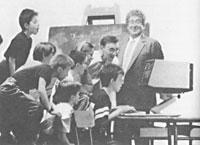You will know that you have to size the matrices. Here I used 2 dimensions in 30 and 40 lines. The number 15 that appears in it limits the number of characters of each variable. In other words, the variables will receive a maximum of 15 characters of the words, so if they are longer they will be saved in the cut memory. (you can change 15 by another number).

This time I want to explain a program to work the matrices.

The program consists of first asking the text, saving it in memory and then inviting it with the original when entering it by the keyboard, indicating the errors.

The program consists of three main parts:

The first from 80 to 170. It is to save the original text, if you click on the space you will not accept it (100 lines of instruction). If you also want to move from the line you are writing to the next one, cancel the remaining variables. (120-140).

Second (1000-1050). It is about correctly displaying the original text on the screen once saved, especially the instruction line 1030. This counts the letters of the variable to write and limits it to real measures, since we must remember that each variable has 15 characters and if the word "that" is entered the variable "that"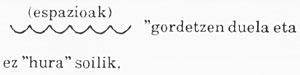Third 200-360. Once deleted enter the same word by word, if you misenter it, when you invite the text Screen with the original, you will be prompted to reenter the word. Line 250 is very important for the program to run well. This makes that if there is any annulled variable in the original array, it also overrides the corresponding z \$ ().

Example:

Once you type the program, clicking on run, you will be asked for the text measures. That is: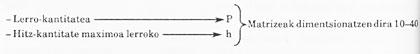We will then enter the original text, for example: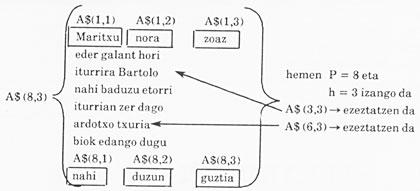The computer has already learned the bertso and now it is our turn.

The screen is deleted and is waiting for the verse we will enter, if the text we enter is different from the original, it will indicate that there is error and ask us to enter it correctly.

 1 Learning verses. J.M. ITURRIOTZ m 1986 5 CLS 10 I NPUT "How many lines do you want to write? "; P 20 INPUT "How many words per line? (maximum)"; h 30 DIM\$ (p, h, 15) 40 DIM z\$ (p, h, 15) 50 LET x = 0 60 FOR 1 = 1 TO p 70 FOR n = 1 TO h 80 I NPUT "; a\$ (1, n) 90 THEN b = a \$ (n) 100 GOT LET a\$ (I, n) = CHR\$ 32 :LOTO 130 150 NEXT n 160 LETx=O 170 NEXT 1 200 PRINT #0; "Write WELL if you have learned";PAUSE 0: CLS: REM. "QUESTIONNAIRE" 210 LETx=O 220 FOR I = 1 TO p 230 FOR n = 1 TO n 240 IF n h THEN GOTO 340 250 LET b = a\$ (l, n): IFG CODE b\$ (1 TO 1) = 32 THEN LET n = n + 1: GOTO 240 260 I NPUT "word"; z\$ (1, n) 270 LET f = 15 280 LET b\$ = z\$11, n) 290 IF CODE b\$ (1 TO 1) = 32 THEN GOTO 260 300 IF (1, n) a\$ (1, n) and FLET to\$ of THB\$ PAUSE 0: GOTO 260 310 IF z\$1I, n) to\$ (1, n) THEN LET f = f - 1: GOTO 300 320 GOSUB 1000 330 NEXT n 340 LET x = 0 350 NEXT I 360 GOTO 200 1000 REM "Screen verse writing subroutine" LET b\$ b\$ (c TO fl: RETURN 1040LET f = f - 1 1050GOT0 1030 Cast the verse!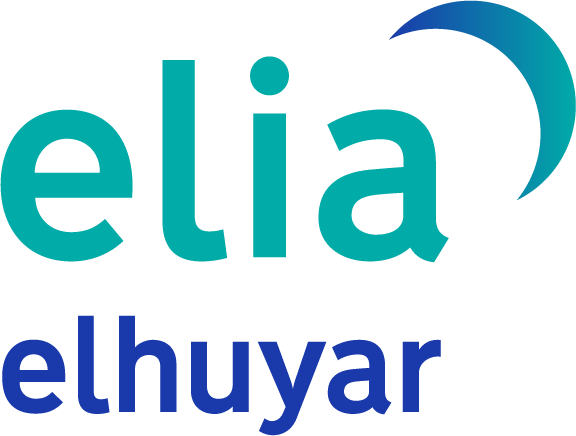eu es fr en ca gl

## Gai honi buruzko eduki gehiago

Elhuyarrek garatutako teknologia

1986-8-1Zientzia